Download Presentation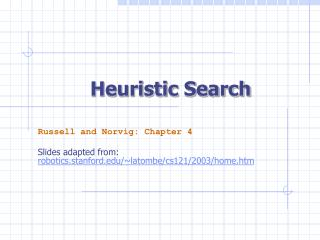Heuristic Search

# Heuristic Search - PowerPoint PPT Presentation

Heuristic Search. Russell and Norvig: Chapter 4 Slides adapted from: robotics.stanford.edu/~latombe/cs121/2003/home.htm. Heuristic Search. Blind search totally ignores where the goals are. A heuristic function that gives us an estimate of how far we are from a goal. Example:I am the owner, or an agent authorized to act on behalf of the owner, of the copyrighted work described.
Download Presentation## Heuristic Search

An Image/Link below is provided (as is) to download presentation

Download Policy: Content on the Website is provided to you AS IS for your information and personal use and may not be sold / licensed / shared on other websites without getting consent from its author.While downloading, if for some reason you are not able to download a presentation, the publisher may have deleted the file from their server.

- - - - - - - - - - - - - - - - - - - - - - - - - - E N D - - - - - - - - - - - - - - - - - - - - - - - - - -
Presentation Transcript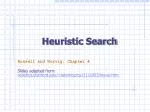### Heuristic Search

Russell and Norvig: Chapter 4

robotics.stanford.edu/~latombe/cs121/2003/home.htm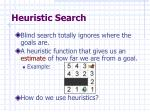Heuristic Search
• Blind search totally ignores where the goals are.
• A heuristic function that gives us an estimate of how far we are from a goal.
• Example:
• How do we use heuristics?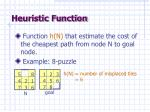1

2

3

4

5

6

7

8

5

8

4

2

1

7

3

6

Heuristic Function
• Function h(N) that estimate the cost of the cheapest path from node N to goal node.
• Example: 8-puzzle

h(N) = number of misplaced tiles = 6

goal

N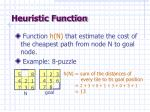1

2

3

4

5

6

7

8

5

8

4

2

1

7

3

6

Heuristic Function
• Function h(N) that estimate the cost of the cheapest path from node N to goal node.
• Example: 8-puzzle

h(N) = sum of the distances of

every tile to its goal position

= 2 + 3 + 0 + 1 + 3 + 0 + 3 + 1

= 13

goal

N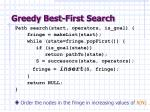Greedy Best-First Search

Path search(start, operators, is_goal) {

fringe = makeList(start);

while (state=fringe.popFirst()) {

if (is_goal(state))

return pathTo(state);

S = successors(state, operators);

fringe = insert(S, fringe);

}

return NULL;

}

• Order the nodes in the fringe in increasing values of h(N)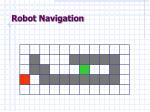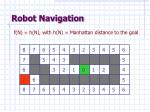8

7

6

5

4

3

2

3

4

5

6

7

5

4

3

5

6

3

2

1

0

1

2

4

7

6

5

8

7

6

5

4

3

2

3

4

5

6

f(N) = h(N), with h(N) = Manhattan distance to the goal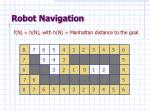f(N) = h(N), with h(N) = Manhattan distance to the goal

8

7

6

5

4

3

2

3

4

5

6

7

5

4

3

5

0

6

3

2

1

0

1

2

4

7

7

6

5

8

7

6

5

4

3

2

3

4

5

6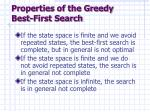Properties of the Greedy Best-First Search
• If the state space is finite and we avoid repeated states, the best-first search is complete, but in general is not optimal
• If the state space is finite and we do not avoid repeated states, the search is in general not complete
• If the state space is infinite, the search is in general not complete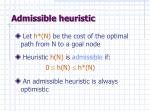• Let h*(N) be the cost of the optimal path from N to a goal node
• Heuristic h(N) is admissible if: 0 h(N)  h*(N)
• An admissible heuristic is always optimistic1

2

3

4

5

6

7

8

5

8

4

2

1

7

3

6

8-Puzzle

N

goal

• h1(N) = number of misplaced tiles = 6 is admissible
• h2(N) = sum of distances of each tile to goal = 13 is admissible
• h3(N) = (sum of distances of each tile to goal) + 3 x (sum of score functions for each tile) = 49 is not admissibleA* Search
• A* search combines Uniform-cost and Greedy Best-first Search
• Evaluation function:f(N) = g(N) + h(N) where:
• g(N) is the cost of the best path found so far to N
• h(N) is an admissible heuristic
• f(N) is the estimated cost of cheapest solution THROUGH N
• 0 <   c(N,N’) (no negative cost steps).
• Order the nodes in the fringe in increasing values of f(N)Completeness & Optimality of A*
• Claim 1: If there is a path from the initial to a goal node, A* (with no removal of repeated states) terminates by finding the best path, hence is:
• complete
• optimal3+3

1+5

2+3

3+4

5+2

0+4

3+2

4+1

2+3

1+3

5+0

3+4

1+5

2+4

8-Puzzle

f(N) = g(N) + h(N)

with h(N) = number of misplaced tiles

goal3+8

2+9

4+7

5+6

3+8

8

7

6

5

4

3

2

3

4

5

6

7+2

7

5

4

3

5

6+1

8+3

6+1

6

3

2

1

0

1

2

4

7+2

7+0

6+1

7

6

5

8+1

6+1

8

7

6

5

4

3

2

3

4

5

6

2+9

3+8

7+2

2+9

3+10

4+5

1+10

4+7

5+4

3+6

2+7

8+3

7+4

5+6

6+3

2+9

3+8

4+5

3+6

2+7

6+5

7+4

0+11

1+10

4+7

3+8

5+6

6+3

7+2

5+4

6+3

f(N) = g(N)+h(N), with h(N) = Manhattan distance to goal

7+0

8+1f(N) = g(N) + h(N), with h(N) = straight-line distance from N to goal

Cost of one horizontal/vertical step = 1

Cost of one diagonal step = 2N

c(N,N’)

N’

h(N)

h(N’)

Consistent Heuristic
• The admissible heuristic h is consistent (or satisfies the monotone restriction) if for every node N and every successor N’ of N:h(N)  c(N,N’) + h(N’)(triangular inequality)1

2

3

4

5

6

7

8

• h1(N) = number of misplaced tiles

5

8

• h2(N) = sum of distances of each tile to goal
• are both consistent

4

2

1

7

3

6

8-Puzzle

N

goalN

c(N,N’)

N’

h(N)

h(N’)

Claims
• If h is consistent, then the function f alongany path is non-decreasing:f(N) = g(N) + h(N) f(N’) = g(N) +c(N,N’) + h(N’) h(N)  c(N,N’) + h(N’) f(N)  f(N’)
• If h is consistent, then whenever A* expands a node it has already found an optimal path to the state associated with this nodeAvoiding Repeated States in A*

If the heuristic h is consistent, then:

• Let CLOSED be the list of states associated with expanded nodes
• When a new node N is generated:
• If its state is in CLOSED, then discard N
• If it has the same state as another node in the fringe, then discard the node with the largest fComplexity of Consistent A*
• Let s be the size of the state space
• Let r be the maximal number of states that can be attained in one step from any state
• Assume that the time needed to test if a state is in CLOSED is O(1)
• The time complexity of A* is O(srlogs)HeuristicAccuracy
• h(N) = 0 for all nodes is admissible and consistent. Hence, breadth-first and uniform-cost are particular A* !!!
• Let h1 and h2 be two admissible and consistent heuristics such that for all nodes N: h1(N)  h2(N).
• Then, every node expanded by A* using h2 is also expanded by A* using h1.
• h2 is more informed than h1
• h2 dominates h1
• Which heuristic for 8-puzzle is better?Iterative Deepening A* (IDA*)
• Use f(N) = g(N) + h(N) with admissible and consistent h
• Each iteration is depth-first with cutoff on the value of f of expanded nodes4

6

8-Puzzle

f(N) = g(N) + h(N)

with h(N) = number of misplaced tiles

Cutoff=44

4

6

6

8-Puzzle

f(N) = g(N) + h(N)

with h(N) = number of misplaced tiles

Cutoff=44

5

4

6

6

8-Puzzle

f(N) = g(N) + h(N)

with h(N) = number of misplaced tiles

Cutoff=45

4

5

4

6

6

8-Puzzle

f(N) = g(N) + h(N)

with h(N) = number of misplaced tiles

Cutoff=46

5

4

5

4

6

6

8-Puzzle

f(N) = g(N) + h(N)

with h(N) = number of misplaced tiles

Cutoff=44

6

8-Puzzle

f(N) = g(N) + h(N)

with h(N) = number of misplaced tiles

Cutoff=54

4

6

6

8-Puzzle

f(N) = g(N) + h(N)

with h(N) = number of misplaced tiles

Cutoff=54

5

4

6

6

8-Puzzle

f(N) = g(N) + h(N)

with h(N) = number of misplaced tiles

Cutoff=54

5

4

7

6

6

8-Puzzle

f(N) = g(N) + h(N)

with h(N) = number of misplaced tiles

Cutoff=54

5

5

4

7

6

6

8-Puzzle

f(N) = g(N) + h(N)

with h(N) = number of misplaced tiles

Cutoff=54

5

5

5

4

7

6

6

8-Puzzle

f(N) = g(N) + h(N)

with h(N) = number of misplaced tiles

Cutoff=54

5

5

5

4

7

6

6

8-Puzzle

f(N) = g(N) + h(N)

with h(N) = number of misplaced tiles

Cutoff=5• Heuristics are intended to orient the search along promising paths
• The time spent computing heuristics must be recovered by a better search
• After all, a heuristic function could consist of solving the problem; then it would perfectly guide the search
• Deciding which node to expand is sometimes called meta-reasoning
• Heuristics may not always look like numbers and may involve large amount of knowledgeWhen to Use Search Techniques?
• The search space is small, and
• There is no other available techniques, or
• It is not worth the effort to develop a more efficient technique
• The search space is large, and
• There is no other available techniques, and
• There exist “good” heuristicsSummary
• Heuristic function
• Greedy Best-first search
• Admissible heuristic and A*
• A* is complete and optimal
• Consistent heuristic and repeated states
• Heuristic accuracy
• IDA*